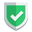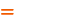我们相信：世界是美好的，你是我也是。平行空间的世界里面，不同版本的生活也在继续...

## 利用`np.zeros()`做加减法

``````import numpy as np
s1 = np.zeros((2, 2), dtype=int) + 2
print(s1, s1.dtype)``````

``````[[2 2]
[2 2]] int32``````

## 利用`np.ones()`做乘法

``````import numpy as np
s1 = np.ones(3, dtype=int) * 2
s2 = np.ones((1, 3)) * 3
print(s1, s1.dtype)
print(s2, s2.dtype)``````

``````[2 2 2] int32
[[3. 3. 3.]] float64``````

## 利用`np.full()`直接填数字/字符

``````import numpy as np
s1 = np.full((1,3),3.14)
print(s1, s1.dtype)``````

``[[3.14 3.14 3.14]] float64``

``````s2 = np.full((1,3),"苏")
print(s2, s2.dtype)``````

``[['苏' '苏' '苏']] <U1``

## 结束语如果本文对您有帮助，或者节约了您的时间，欢迎打赏瓶饮料，建立下友谊关系。本博客不欢迎：各种镜像采集行为。请尊重原创文章内容，转载请保留作者链接。【福利】 腾讯云最新爆款活动！1核2G云服务器首年50元！【源码】本文代码片段及相关软件，请点此获取更多信息【绝密】秘籍文章入口，仅传授于有缘之人python